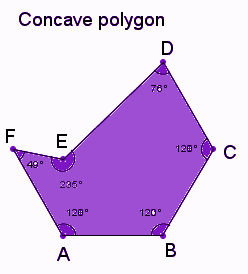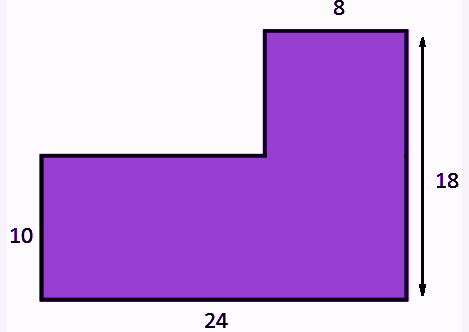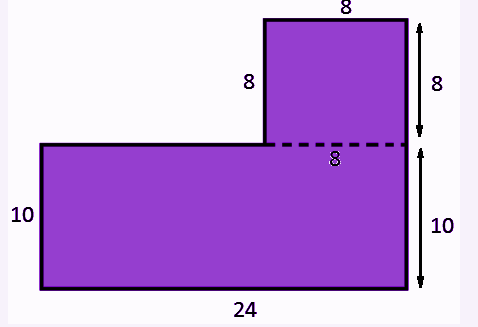# Concave Polygon

A Concave polygon is a polygon that has at least one interior angle greater than 180 degrees. It must have at least four sides. The shape of the concave polygon is usually irregular. A concave polygon is a polygon which is not convex. This polygon is just the opposite of a convex polygon

A simple polygon is considered as a concave polygon if and only if at least one of the interior angles is a reflex angle (between 180° and 360°). It is also called a non-convex polygon. The area and perimeter of it will depend on the shape of the particular polygon.

## Concave Polygon Definition

A polygon has at least one angle that measures more than 180 degrees, which is called a concave polygon. The vertices (endpoints) of this polygon are inwards as well as outwards. They are just the opposite of the convex polygons. A triangle cannot be considered as a concave polygon because it has only three sides and whose sum of interior angles is 180 degrees.## Irregular Concave Polygon

A polygon may be either convex or concave polygon. Those polygons are further classified into regular or irregular. The irregular polygon can have sided with different measures and also each interior angles measures are also varied. It is noted that all the concave polygons are irregular since the interior angles of the polygon are of different measures.

Is Star a Concave Polygon?

Yes, a star is a concave polygon. Because concave polygon should have at least 4 sides. Also, one or more interior angles should be greater than 180 degrees.

## Regular Concave Polygon

A regular polygon is a polygon where the length of each side is the same and all the interior angles are equal. By the definition of a concave polygon, it contains at least one of the interior angles more than 180 degrees. Also, the sum of the interior angles of a polygon is (n – 2) x 180, where n is the number of sides. So, it is not possible to have a polygon with all sides equal and an angle greater than 180 degrees. Hence, regular polygons are never concave.

## Concave Polygon Formulas

Let us discuss the formulas such as area and the perimeter of the concave polygon below.

### Area of a Concave Polygon

Unlike a regular polygon, there is no easy formula to find the area of a concave polygon. Each side could be of a different length, and each interior angle could be different. So, we have to split the concave polygon into triangles or parallelograms or other shapes for which we can easily find the area.

Area of Concave Polygon = Area of the different shapes available in it

### The perimeter of a Concave Polygon

The perimeter of any polygon is defined as the total distance covered around the boundary of the polygon. Similarly, the perimeter of a concave polygon is defined as the total distance covered around the boundary of the concave polygon. The perimeter of a concave polygon can be found by adding together the length of all the sides.

The perimeter of Concave Polygon = Sum of all its sides

## Concave Polygon Properties

The following are some of the important properties of a concave polygon:

• A concave polygon has at least one vertex that points inwards to give the concave shape
• It has at least one reflex angle. It means that at least one of the interior angles is greater than 180° and less than 360°
• If a line segment is drawn crossing the concave polygon, it will intersect the boundary more than two times
• A polygon can have more than one diagonal that lie outside the boundary
• A concave polygon has at least one pair of sides joining a vertex that goes outside the vertex
• We can divide a concave polygon into a set of convex polygons
• A triangle cannot be a concave polygon

### Exterior Angle of a Concave Polygon

The exterior angles of a polygon always add up to 3600. A concave polygon has one or more of its vertices “pushed inside”. Hence, they point towards the interior of the polygon

### Interior Angle of a Concave Polygon

The interior angles of any polygon always add up to a constant value, which depends only on the number of sides of the polygon. For example, the interior angles of a pentagon always add up to 5400, no matter if it is convex or concave, or what size and shape it is. The sum of the interior angles formula of a polygon is given by:

Sum of interior angles = 180 * (n – 2) degrees

where n is the number of sides.

• Square: n =4; sum of interior angles = 180 x (4-2) = 360 degrees
• Pentagon: n = 5; sum of interior angles = 180 x (5-2) = 540 degrees
• Hexagon: n = 6; sum of interior angles = 180 x (6-2) = 720 degrees

### Solved Examples

Question:

Find the area and perimeter for the concave polygon given below:Solution:

In this figure, one of the shapes is a rectangle and the other one is a square.First, find the area of rectangle and square and then add the two areas to get the total area of a concave polygon.

Step 1: Find the area of the rectangle?Area of the rectangle = length x widthHere, length = 24 and width = 10Area = 24 x 10 = 240 sq units

Step 2: Find the area of the square?Area of square = Side x Side

Side = 8Area = 8 x 8 = 64 sq units

Step 3: Total area of the concave polygon = Area of rectangle + Area of square

TA = 240 + 64

TA = 304 sq units.

Step 4: Perimeter of given polygon = Sum of all sides

P = 24 + 18 + 8 + 8 + 16 + 10

P = 84 units.

Question 2: If the side lengths of a concave polygon are 10 cm, 11 cm, 12 cm, 13 cm, 14 cm, 16 cm. What is its perimeter?

Solution: Given, sides of the polygon are 10 cm, 11 cm, 12 cm, 13 cm, 14 cm, 16 cm.

Perimeter = sum of all sides

Perimeter = 10+11+12+13+14+16 = 76 cms.

Stay tuned with BYJU’S – The Learning App and download the app to learn all the important Maths-related articles to learn with ease.

## Frequently Asked Questions – FAQs

### What is meant by a concave polygon?

A concave polygon is a polygon that has at least one of its interior angles greater than 180 degrees.

### How many sides does a concave polygon have?

A concave polygon has at least four sides.

### What is the difference between concave and convex polygon?

A convex polygon has all its interior angles less than 180 degrees whereas a concave polygon has at least one interior angle more than 180 degrees.

### How to find the area of a concave polygon?

Divide the concave polygon into separate triangles, by drawing diagonal lines from one vertex point to other vertices. Now find the area of all the triangles and add them all to get the final area.

### What are the other types of a polygon?

The types of polygons are:
Regular Polygons
Irregular Polygons
Concave Polygons
Convex Polygons
Trigons